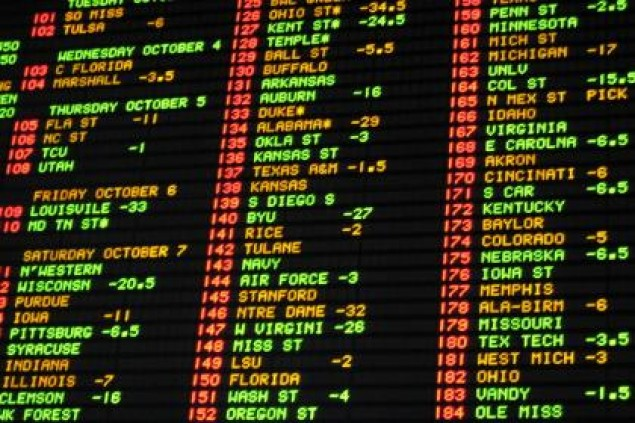# Calculating Return on Investment (ROI) in Sports Betting##### Calculating Return on Investment (ROI) in Sports Betting

Return on investment is a performance measure used to evaluate the efficiency of an investment. To calculate ROI, the return of an investment (or in this case, the profit earned from your sports betting system) is divided by the cost of the investment with the result typically being expressed on this website as a percentage.

ROI is perhaps the best way to analyze the success of a betting system and, for this example, we will assume a risk of \$100 on each bet. We will start by taking your net profit and dividing it by the total risk. For example, if you created a system that had 500 games played and you won 25 units off of it, your ROI would be calculated thusly: (25 units X \$100) / (500 games X \$100) = .05. This number is typically viewed as a percentage, so this system would have a return on investment of 5%. Essentially we have taken the gains from our bets and then divided that by the total cost of investment — or the amount of money we have put at risk.

One of the reasons this number is so important is that is helps us determine if a system is truly profitable. In baseball, there are often systems with losing records because we take to many underdogs with plus money. This means that we can not use winning percentage as a metric of success, but we can determine our return on investment by dividing our units earned by the number of games we have wagered on.

##### David Solar

David was the Content Manager at Sports Insights. He has since moved on to greener pastures.

• ###### Evan Feldman
05/04/2013 at 5:35 pm

I think you vastly understate your ROI. In your example, the amount you really risk is the amount of capital you started with. For example, if you started the season with \$10,000 and risked \$100 per game, you would have a return of 25%. If you went broke, you would have lost \$10,000, the amount you actually risked. You didn’t really risk \$50,000, because you didn’t start with that. I do not believe your cumulative bet amount should be the actual amount you risked on your system. Your example is telling me that I earned 5% on my investment but that’s just not true. It could be true, or it could be much higher. It all depends on the amount you started with. I think a better example of ROI is saying you bet 1% of your bankroll on each pick, as opposed to \$100, which would have given you a 25% return. That percentage per pick could be adjusted to suit the individual and give an actual return on their investment.

• ###### PJ
05/07/2013 at 3:05 pm

Hey Evan,

Using your example of a \$10,000 bankroll, if you only make four wagers of \$100, your \$10,000 was never invested and at-risk, only \$400 was actually invested.

Whether your bankroll is \$10,000 or \$1,000,000, your ROI is still the same if you only make four bets of \$100.

You’re thinking interest rate, not return on investment.

• ###### Evan
05/09/2013 at 1:34 pm

• ###### PJ
05/14/2013 at 8:33 am

This should help, here is the formula for calculating return on investment used in the financial world. It has nothing to do with starting bankroll or capital.

Or that matters is what you actually invested (or wagered) and how much you earned.

http://www.investopedia.com/terms/r/returnoninvestment.asp

Also, here is a good forum thread that explains this further:

• ###### PJ
05/14/2013 at 8:37 am

And for the record, if you read our other betting against the public articles, we don’t use ROI as the only factor in judging a system. For the most part, we always recommend following units won as the best way to determine success. However, units won is not the end all, be all. Especially when you can improve ROI by reducing the number of wagers needed to produce of profit of similar units.

• ###### Paid Football Betting Tips Archive
07/16/2014 at 9:46 am

Hi PJ,

Nice article! but I have similar problem in this ROI math – I am not as good as other with this ROI stuff – that I have started to test my development and experiments about football betting tips by myself. After I read your article above I am not sure about my results archive ( check my link on name).

In real example on June 2014 archive :
* 10 unit per pick.
PERFORMANCE – PICKS – UNIT
WIN – 44.00 – 440
WIN HALF – 8.00 – 40
PUSH DRAW – 1.00 – 10
LOSE – 35.00 – 350
LOSE HALF – 2.00 – 10
TOTAL PICKS – 90.00 – 900
AVERAGE ODDS 1.91

I have calculated my ROI based on ;

1. Total Winning Units – Total Lost Units = Profit Unit (it could be positive or negatif)
Total WIN 480 unit
Total Lose 360 unit
Profit Unit= +120 unit

2. Then Profit Unit : Total Unit Stake = XX.XX
120 : 900 = 0.13

3. To convert XX.XX into percentage it should be multiplied by 100 = %ROI%
0.13 x 100 = 13.3%

I assume it reasonable that ROI stuff on above case actually WIN in unit with positive returns.